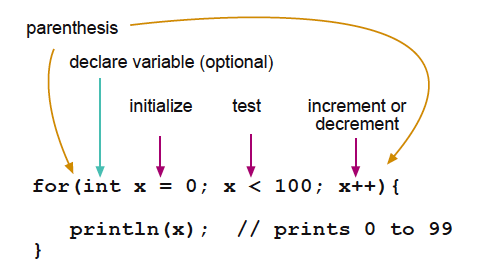## Introduction to Programming

In this workshop, we use processing.

Binary and Decimal
We use decimal system in our everyday life when dealing with numbers. Decimal is a system that uses unit of Ten or another words base as Ten. On the other hand, Binary system uses two as a base, in another words base as two.Bits and Bytes
A bit stores just a 0 or 1, and it is the smallest unit of storage
“In the computer it’s all 0’s and 1’s” … computer is “thinking” in the world of bits-binary
One byte = grouping of 8 bits

Q. What is the biggest number you can express with 1 byte? 255

Data Type
boolean (0,1) >> 1 bit
byte (0-255) >> 8 bits / 1 byte
int (-32,768 to 32,767) >> 16 bit/ 2 bytes
unsigned int (0 to 65,535) >> 16 bits/ 2 bytes
long (-2,147,483,648 to 2,147,483,647) >> 32 bits/ 4 bytes
float (as large as 3.4028235E+38 and as low as -3.4028235E+38) >> 32 bits/ 4 bytes
char ( ‘A’, ‘B’, ‘C’) >> 8 bits/ 1 byte

Variables
A variable is a place to store a piece of data. It has a name, a value, and a type

exercise:
Let’s open processing and open new sketch.
Create one variable with the name you want, data type int.
Let’s give initial value as 0

tip: In Processing and Arduino language, you need “;” (semicolon) to end a statement. You can remember it like the game “Simon says..” If you want the program to do something, you need to say “Simon says” >> end with ;

Array
An array is a collection of variables that are accessed with an index number. You can imagine variable as 1 box of storage and array as a shelf of storage with many boxes. The boxes are numbered so you need to say which box of the which shelf you store the number or take the number out from.

int name_of_the_array ;
int name_of_the_array []={0,0,0,0,0};

function
In programming, a named section of a program that performs a specific task. In this sense, a function is a type of procedure or routine. Some programming languages make a distinction between a function, which returns a value, and a procedure, which performs some operation but does not return a value.

Most programming languages come with a pre-written set of functions that are kept in a library. You can also write your own functions to perform specialized tasks.

from http://www.webopedia.com/TERM/F/function.html

exercise:
In processing library, there is a function called print(). This will print what is in the () to print in the console screen (the black screen on the bottom). Let’s try to print the variable you’ve created.

tip: In Processing and Arduino, there are some fixed functions that are used in the sketches. In processing it is void draw() function, and in Arduino it is void loop() function, that serves as the main loop. also both of them uses void setup() function that is called only once when the sketch runs.

exercise:
add draw function and place the print function in it. What happens?

exercise:
count up the variable you’ve created in the draw function. Can you see the incrementing number on the screen?

tip: A = A + 1

exercise:
Make your own function. Create a function that does the calculation you’ve just added in draw function. This new function should be written outside of the draw function. Then call this function in draw function.

if
if, which is used in conjunction with a comparison operator, tests whether a certain condition has been reached, such as an input being above a certain number. (from Arduino.cc)

if (the condition written here is true) then { do the thing written here }

exercise:
if ( you == student)
{ raise_your_right_hand();
if (you == female){
stand_up();
if (your_age < 25){ turn_right(); } else { turn_left(); } } else { raise_your_left_hand(); } } smile(); >> what are you doing now? are you standing? sitting? smiling?

comparison operators ==, !=, <, >
if (A == B) –if A is the same as B
if (A != B) –if A is not the same as B
if (A < B) --if A is smaller than B if (A > B) –if A is bigger than B

and you can make combinations
if (A == B && B == C) –if A is the same as B and B is the same as C
if (A == B || B == C) — if A is the same as B or B is the same as C

exercise:
Navigating Felix.
He needs to go from the sewing machine corner to the door. He can take only one step per “loop”. How can we instruct him to go to the door and get out of the room?

void draw(){
Take_one_step();

}

for
The for statement is used to repeat a block of statements enclosed in curly braces. An increment counter is usually used to increment and terminate the loop. The for statement is useful for any repetitive operation, and is often used in combination with arrays to operate on collections of data/pins.
(from Arduino.cc)exercise:
Let’s draw ten ellipse!
if you type ellipse(5,50,10,10); it will draw ellipse size of 10by10 at the position of x coordinate 5, y coordinate 50.

Now, can we draw 10 ellipse on the campus? you can chose any size and position.
for (int i=0; i<10; i++){ ellipse(10*i+5,50,10,10); }

“for” is very useful when you are using arrays.
For example, you want to reset all your array’s number as 0. Let’s say your array called elab[] was an array of twenty, Then you can write as:
for (int i=0; i<20; i++){ elab[i]=0; }